A gas that follows Boyle’s law, Charle’s law, Avogadro law strictly, is called an ideal gas. Real gases follow these laws only under certain specific condition. When forces of interaction are practically negligible.

## Ideal Gas Equation

The above laws can be combined together in a single equation which is known as ideal gas equation.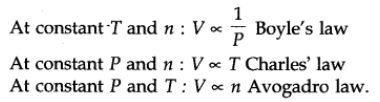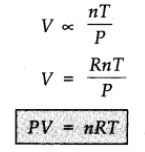where R is proportionality constant. On rearranging the equation, we obtain

pV = n RT

This equation is called ideal gas equation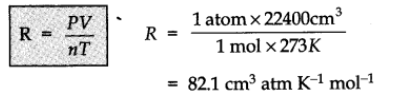R is called gas constant. It is same for all gases. Therefore, it is also called universal gas constant.

Values of R:

• R = 8.314 J K-1 mol-1
• R = 0.0821 L atm K-1 mol-1
• R = 2 cal K-1 mol-1.

If temperature, volume and pressure of a fixed amount of gas vary from T1, V1 and p1 to T2, V2 and p2 then we can write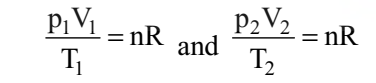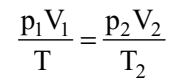This equation is also known as combined gas law.

## Variation of the Ideal Gas Equation

Ideal gas equation can be rearranged as follows: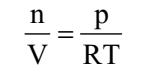Replacing n by m/M, we get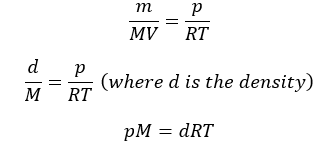## Dalton’s law of Partial Pressures

It states that the total pressure exerted by the mixture of non-reactive gases is equal to the sum of the partial pressures of individual gases i.e., the pressures which these gases would exert if they were enclosed separately in the same volume and under the same conditions of temperature. In a mixture of gases, the pressure exerted by the individual gas is called partial pressure.

Mathematically,

Ptotal = p1 + p2 + p3 + …… (at constant T, V)

where ptotal is the total pressure exerted by the mixture of gases and p1, p2, p3 etc. are partial pressures of gases.

### Aqueous tension

Gases are generally collected over water and therefore are moist. Pressure of dry gas can be calculated by subtracting vapour pressure of water from the total pressure of the moist gas which contains water vapours also. Pressure exerted by saturated water vapour is called aqueous tension.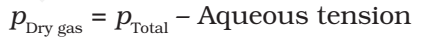### Partial pressure in terms of mole fraction

Suppose at the temperature T, three gases, enclosed in the volume V, exert partial pressure p1, p2 and p3 respectively.

Then,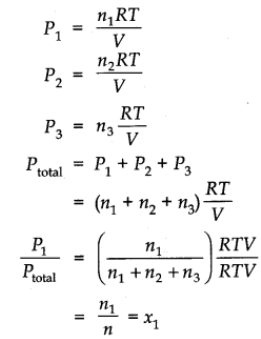where,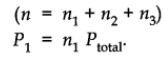x1 is called mole fraction of first gas.

Thus, p1 = x1 ptotal

Similarly, for other two gases we can write

p2 = x2 ptotal and p3 = x3 ptotal

Thus, a general equation can be written as

pi = xi ptotal

where pi and xi are partial pressure and mole fraction of ith gas respectively.